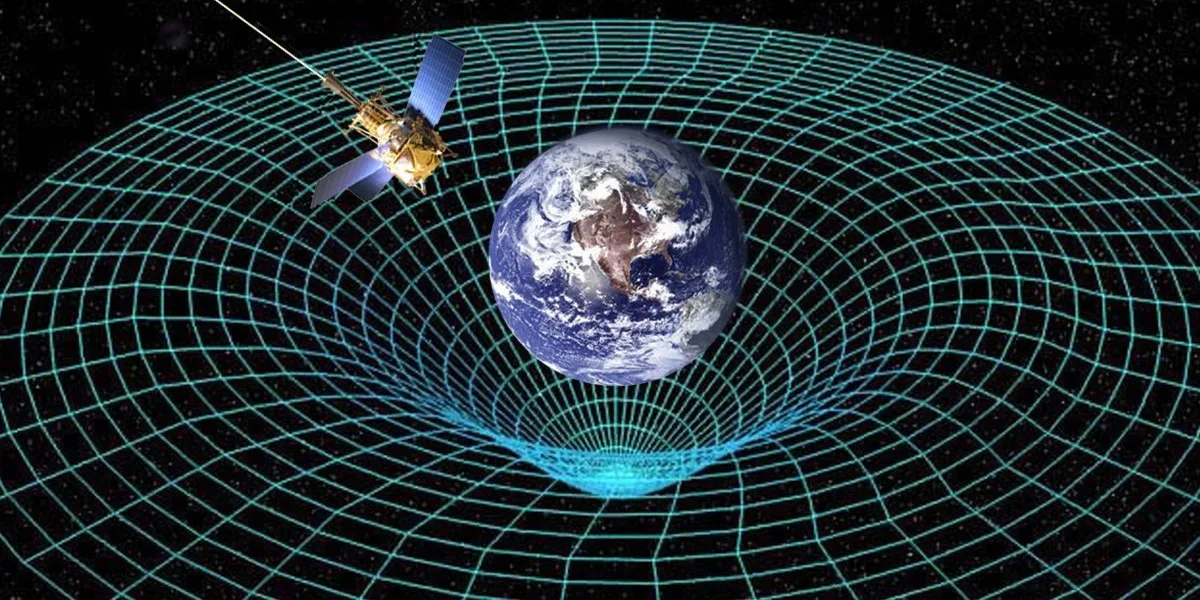Science

# Einstein’s Theory of General Relativity Was Confirmed in a Distant Galaxy

Michael Koppitz (Max Planck Institute for Gravitational Physics (Albert Einstein Institute)/Zuse Institute Berlin)

Albert Einstein’s theory of relativity revolutionized how we understand space, time, and gravity. While most of us can only boast a surface-level understanding of the theory, it’s time to brush up on the game-changing concept, seeing as a new study was able to validate Einstein’s theory of general relativity.

Led by Dr. Thomas Collett of the Institute of Cosmology and Gravitation at the UK’s University of Portsmouth, an international team of astronomers conducted what they say was the first test of general relativity on a large astronomical scale. “A Precise Extragalactic Test of General Relativity,” which was published in the journal Science on Thursday, found that gravity’s behavior in distant galaxies reflects that way gravity behaves in our solar system, just as Einstein’s theory predicted.Einstein determined that distortions in spacetime are felt as gravity.Michael Koppitz (Max Planck Institute for Gravitational Physics (Albert Einstein Institute)/Zuse Institute Berlin)

So what is the theory of general relativity? We first have to look at the physicist’s theory of special relativity, which Einstein introduced in his 1905 paper “On the Electrodynamics of Moving Bodies.” This is where Einstein first calculated the speed of light (186,000 miles per second) and posited that the laws of physics appear the same to all observers. These two principles would form the basis of special relativity, where Einstein deduced, in its simplest terms, that everything is moving relative to everything else.

Einstein’s theory of special relativity lays the groundwork for his 1915 theory of general relativity and its equivalence principle of observing gravity. From there, Einstein explores the ways in which spacetime, a mathematical model that joins space and time in a continuum, is curved by the presence of gravity, matter, energy, and momentum. It’s a geometric theory of how gravitation affects the energy that moves matter around and can be used to predict how much curvature is created by a mass.

To validate these tenets of general relativity, Collett’s team used data from NASA’s Hubble Space Telescope and a technique called gravitational lensing, whereby a massive object acts like a lens by bending light so that the image of the background object is distorted. In this scenario, the researchers used galaxy ESO 325-G004 as their lens, which is roughly 500 million light-years from Earth.

The galaxy serving as a lens allowed Collett to measure the mass and curvature of the spacetime in the neighboring galaxy. By comparing the galaxy’s mass with the measured curvature of spacetime, the team found the mass of the galaxy to be what the theory of general relativity predicts.

While the team plans to study the spacetime curvature of other galaxies, their groundbreaking first study verifies that Einstein’s theory of general relativity can apply to matter outside of our solar system. Not only does this vindicate some long-held theories, it could be a boon for cosmologists in their quest to identify the location and characteristics of other galaxies.

Related Tags
Share: